Home > CC3 > Chapter Ch10 > Lesson 10.1.5 > Problem10-68

10-68.
1. Simplify each of the following expressions. Homework Help ✎

1. (5x3)2

2.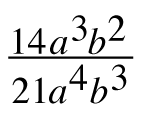3. 2m3n2 · 3mn4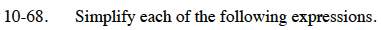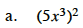Notice that when you put exponents outside of a pair of parentheses, the exponent applies to all of the factors within the parentheses.

$5^2{\it x}^{3\left(2\right)}$

$5^2{\it x}^6$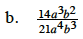Look for Giant Ones.

$\frac{2}{3ab}$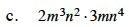When multiplying the same bases together, add the exponents.